Ex 3.1

Chapter 3 Class 7 Data Handling
Serial order wise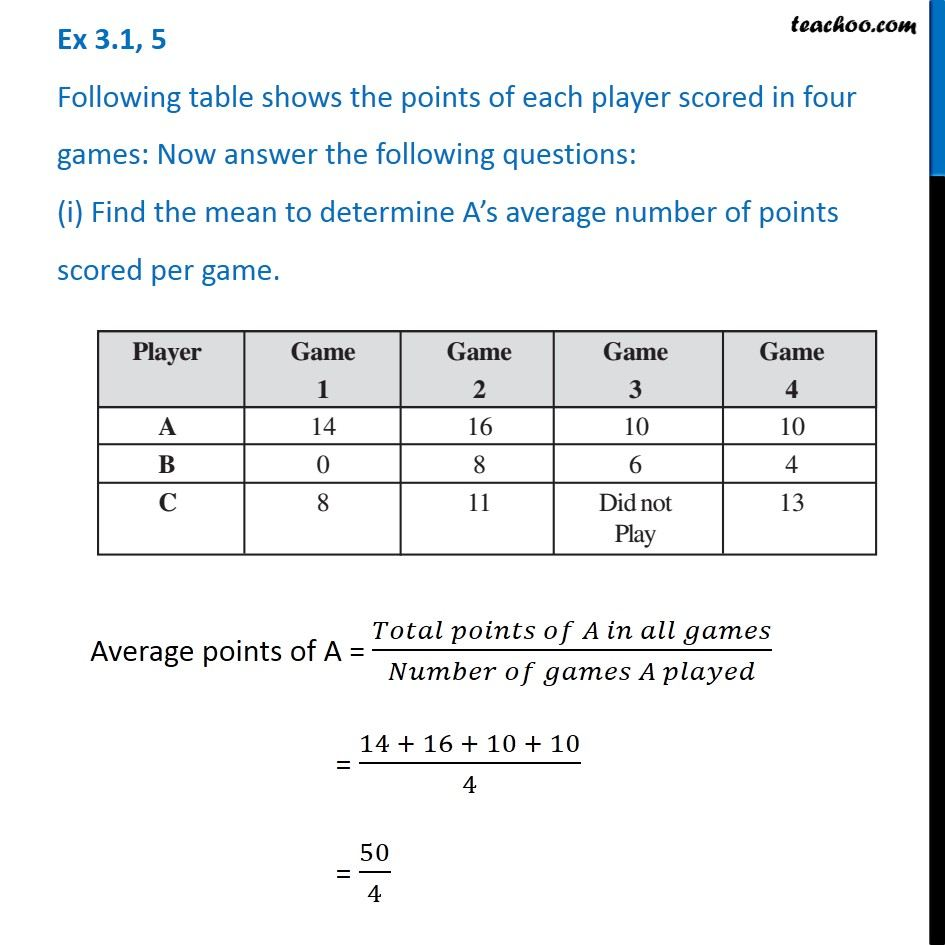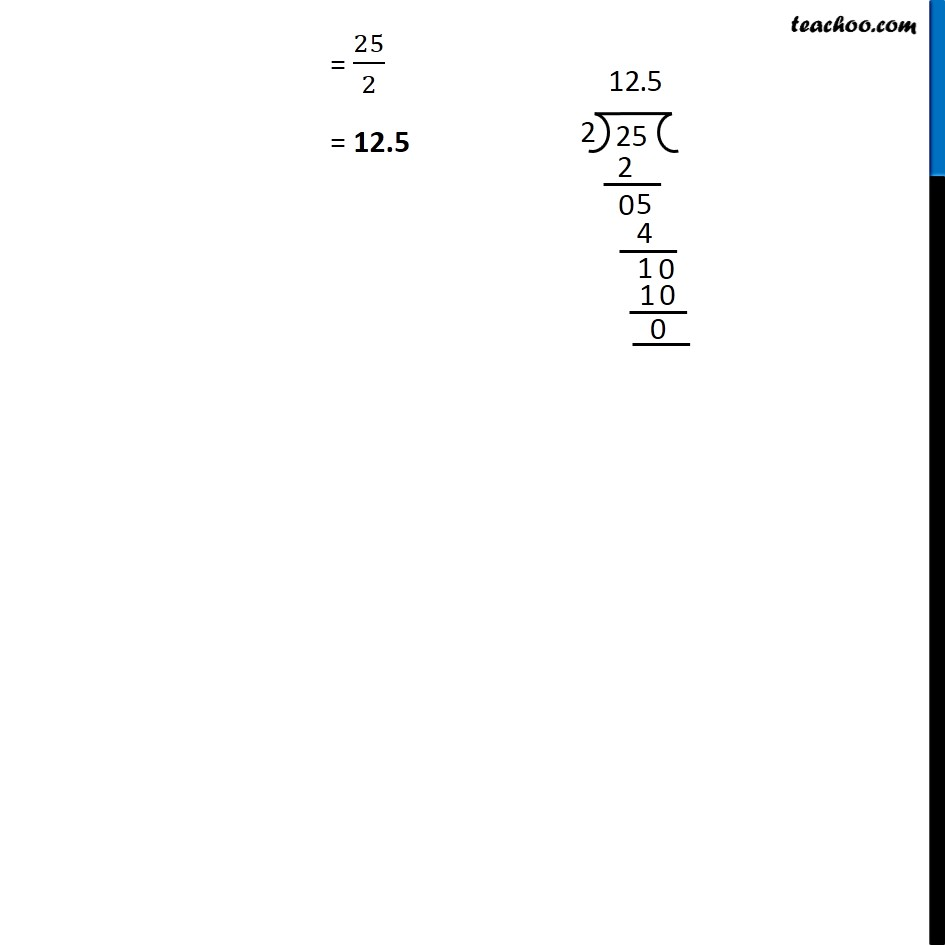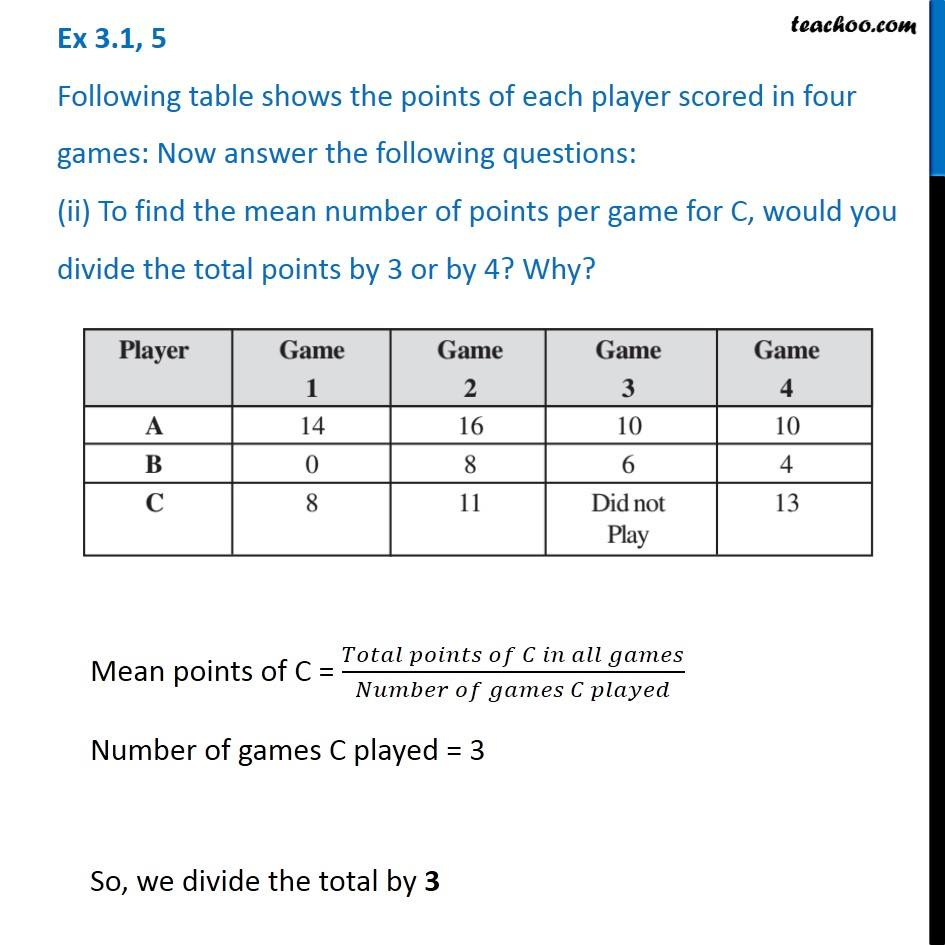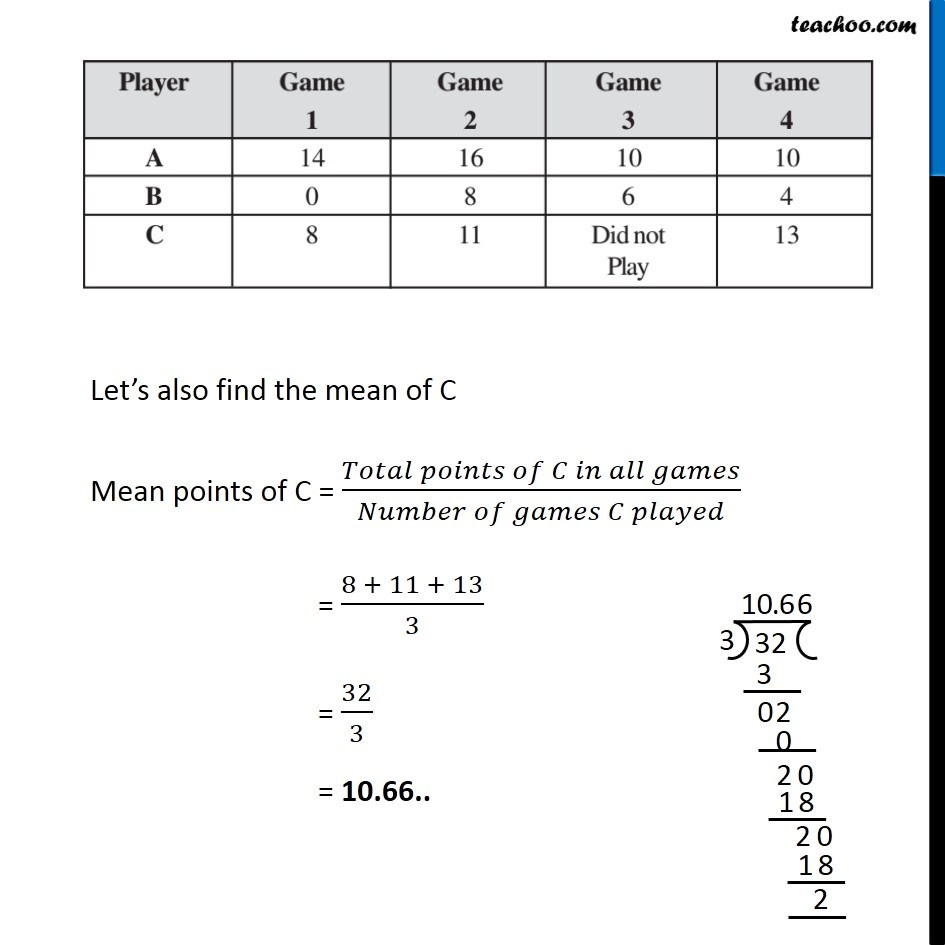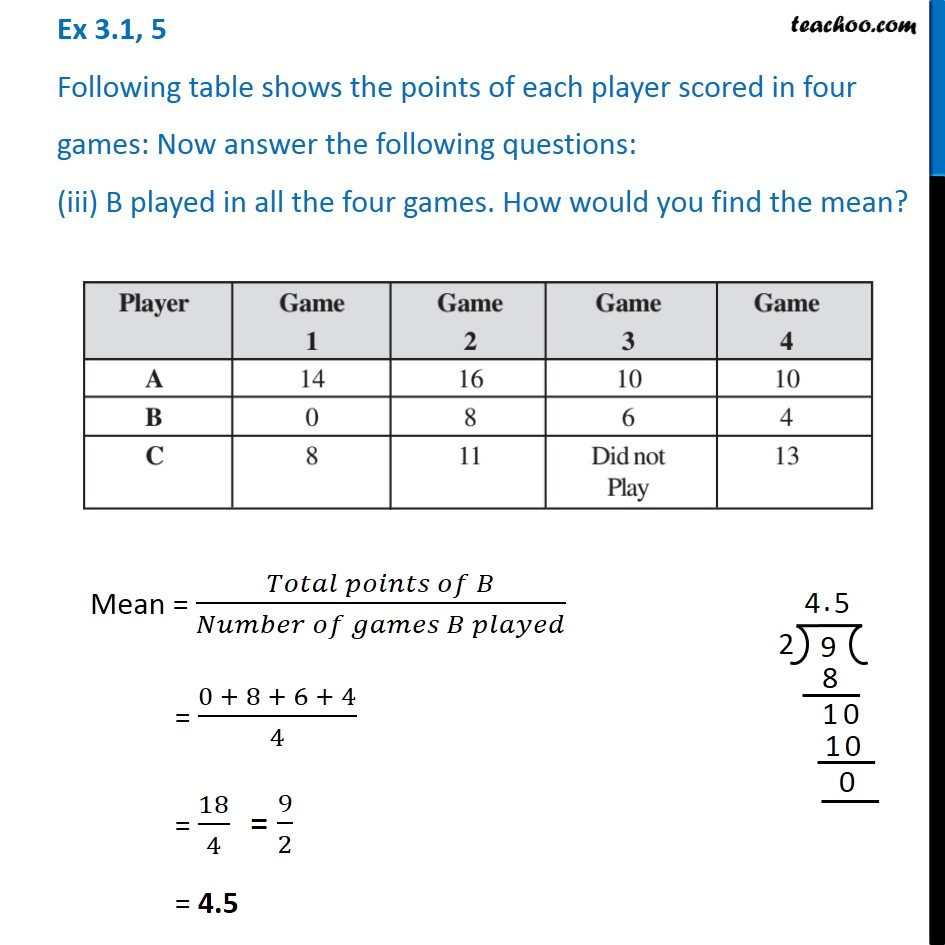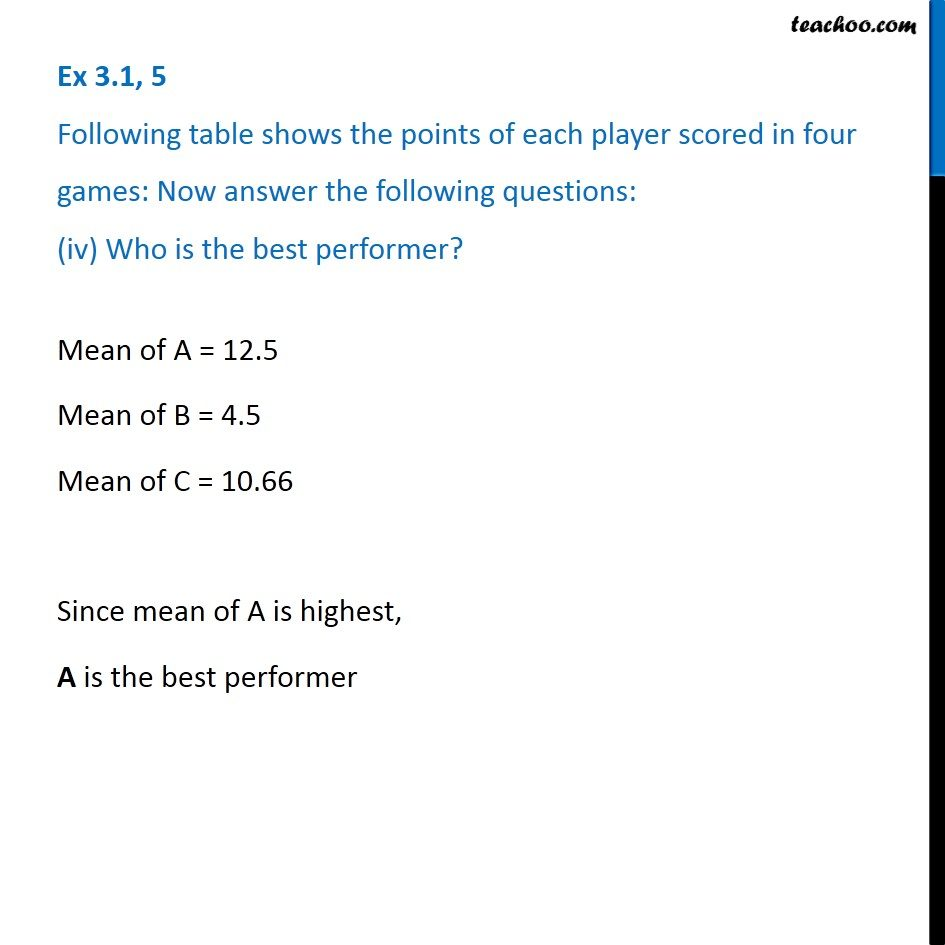Learn in your speed, with individual attention - Teachoo Maths 1-on-1 Class

### Transcript

Ex 3.1, 5 Following table shows the points of each player scored in four games: Now answer the following questions: (i) Find the mean to determine A’s average number of points scored per game. Average points of A = (𝑇𝑜𝑡𝑎𝑙 𝑝𝑜𝑖𝑛𝑡𝑠 𝑜𝑓 𝐴 𝑖𝑛 𝑎𝑙𝑙 𝑔𝑎𝑚𝑒𝑠)/(𝑁𝑢𝑚𝑏𝑒𝑟 𝑜𝑓 𝑔𝑎𝑚𝑒𝑠 𝐴 𝑝𝑙𝑎𝑦𝑒𝑑) = (14 + 16 + 10 + 10)/4 = 50/4 = 25/2 = 12.5 Ex 3.1, 5 Following table shows the points of each player scored in four games: Now answer the following questions: (ii) To find the mean number of points per game for C, would you divide the total points by 3 or by 4? Why? Mean points of C = (𝑇𝑜𝑡𝑎𝑙 𝑝𝑜𝑖𝑛𝑡𝑠 𝑜𝑓 𝐶 𝑖𝑛 𝑎𝑙𝑙 𝑔𝑎𝑚𝑒𝑠)/(𝑁𝑢𝑚𝑏𝑒𝑟 𝑜𝑓 𝑔𝑎𝑚𝑒𝑠 𝐶 𝑝𝑙𝑎𝑦𝑒𝑑) Number of games C played = 3 So, we divide the total by 3 Let’s also find the mean of C Mean points of C = (𝑇𝑜𝑡𝑎𝑙 𝑝𝑜𝑖𝑛𝑡𝑠 𝑜𝑓 𝐶 𝑖𝑛 𝑎𝑙𝑙 𝑔𝑎𝑚𝑒𝑠)/(𝑁𝑢𝑚𝑏𝑒𝑟 𝑜𝑓 𝑔𝑎𝑚𝑒𝑠 𝐶 𝑝𝑙𝑎𝑦𝑒𝑑) = (8 + 11 + 13)/3 = 32/3 = 10.66.. Ex 3.1, 5 Following table shows the points of each player scored in four games: Now answer the following questions: (iii) B played in all the four games. How would you find the mean?Mean = (𝑇𝑜𝑡𝑎𝑙 𝑝𝑜𝑖𝑛𝑡𝑠 𝑜𝑓 𝐵)/(𝑁𝑢𝑚𝑏𝑒𝑟 𝑜𝑓 𝑔𝑎𝑚𝑒𝑠 𝐵 𝑝𝑙𝑎𝑦𝑒𝑑) = (0 + 8 + 6 + 4)/4 = 18/4 = 4.5 Ex 3.1, 5 Following table shows the points of each player scored in four games: Now answer the following questions: (iv) Who is the best performer? Mean of A = 12.5 Mean of B = 4.5 Mean of C = 10.66 Since mean of A is highest, A is the best performer